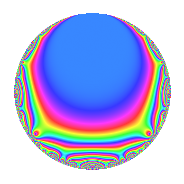# Properties

 Label 2008.1.c.bLevel 2008 Weight 1 Character orbit 2008.c Self dual Yes Analytic conductor 1.002 Analytic rank 0 Dimension 3 Projective image $$D_{7}$$ CM disc. -2008 Inner twists 2

# Related objects

## Newspace parameters

 Level: $$N$$ = $$2008 = 2^{3} \cdot 251$$ Weight: $$k$$ = $$1$$ Character orbit: $$[\chi]$$ = 2008.c (of order $$2$$ and degree $$1$$)

## Newform invariants

 Self dual: Yes Analytic conductor: $$1.00212254537$$ Analytic rank: $$0$$ Dimension: $$3$$ Coefficient field: $$\Q(\zeta_{14})^+$$ Coefficient ring: $$\Z[a_1, \ldots, a_{7}]$$ Coefficient ring index: $$1$$ Projective image $$D_{7}$$ Projective field Galois closure of 7.1.8096384512.1 Artin image size $$14$$ Artin image $D_7$ Artin field Galois closure of 7.1.8096384512.1

## $q$-expansion

Coefficients of the $$q$$-expansion are expressed in terms of a basis $$1,\beta_1,\beta_2$$ for the coefficient ring described below. We also show the integral $$q$$-expansion of the trace form.

 $$f(q)$$ $$=$$ $$q$$ $$+ q^{2}$$ $$+ q^{4}$$ $$-\beta_{1} q^{7}$$ $$+ q^{8}$$ $$+ q^{9}$$ $$+O(q^{10})$$ $$q$$ $$+ q^{2}$$ $$+ q^{4}$$ $$-\beta_{1} q^{7}$$ $$+ q^{8}$$ $$+ q^{9}$$ $$+ \beta_{2} q^{11}$$ $$-\beta_{1} q^{14}$$ $$+ q^{16}$$ $$+ ( -1 + \beta_{1} - \beta_{2} ) q^{17}$$ $$+ q^{18}$$ $$+ ( -1 + \beta_{1} - \beta_{2} ) q^{19}$$ $$+ \beta_{2} q^{22}$$ $$+ \beta_{2} q^{23}$$ $$+ q^{25}$$ $$-\beta_{1} q^{28}$$ $$+ ( -1 + \beta_{1} - \beta_{2} ) q^{29}$$ $$+ ( -1 + \beta_{1} - \beta_{2} ) q^{31}$$ $$+ q^{32}$$ $$+ ( -1 + \beta_{1} - \beta_{2} ) q^{34}$$ $$+ q^{36}$$ $$-\beta_{1} q^{37}$$ $$+ ( -1 + \beta_{1} - \beta_{2} ) q^{38}$$ $$+ \beta_{2} q^{41}$$ $$-\beta_{1} q^{43}$$ $$+ \beta_{2} q^{44}$$ $$+ \beta_{2} q^{46}$$ $$+ ( 1 + \beta_{2} ) q^{49}$$ $$+ q^{50}$$ $$+ \beta_{2} q^{53}$$ $$-\beta_{1} q^{56}$$ $$+ ( -1 + \beta_{1} - \beta_{2} ) q^{58}$$ $$-\beta_{1} q^{59}$$ $$+ \beta_{2} q^{61}$$ $$+ ( -1 + \beta_{1} - \beta_{2} ) q^{62}$$ $$-\beta_{1} q^{63}$$ $$+ q^{64}$$ $$+ ( -1 + \beta_{1} - \beta_{2} ) q^{68}$$ $$+ q^{72}$$ $$-\beta_{1} q^{73}$$ $$-\beta_{1} q^{74}$$ $$+ ( -1 + \beta_{1} - \beta_{2} ) q^{76}$$ $$+ ( -1 - \beta_{2} ) q^{77}$$ $$+ ( -1 + \beta_{1} - \beta_{2} ) q^{79}$$ $$+ q^{81}$$ $$+ \beta_{2} q^{82}$$ $$-\beta_{1} q^{86}$$ $$+ \beta_{2} q^{88}$$ $$-\beta_{1} q^{89}$$ $$+ \beta_{2} q^{92}$$ $$+ ( 1 + \beta_{2} ) q^{98}$$ $$+ \beta_{2} q^{99}$$ $$+O(q^{100})$$ $$\operatorname{Tr}(f)(q)$$ $$=$$ $$3q$$ $$\mathstrut +\mathstrut 3q^{2}$$ $$\mathstrut +\mathstrut 3q^{4}$$ $$\mathstrut -\mathstrut q^{7}$$ $$\mathstrut +\mathstrut 3q^{8}$$ $$\mathstrut +\mathstrut 3q^{9}$$ $$\mathstrut +\mathstrut O(q^{10})$$ $$3q$$ $$\mathstrut +\mathstrut 3q^{2}$$ $$\mathstrut +\mathstrut 3q^{4}$$ $$\mathstrut -\mathstrut q^{7}$$ $$\mathstrut +\mathstrut 3q^{8}$$ $$\mathstrut +\mathstrut 3q^{9}$$ $$\mathstrut -\mathstrut q^{11}$$ $$\mathstrut -\mathstrut q^{14}$$ $$\mathstrut +\mathstrut 3q^{16}$$ $$\mathstrut -\mathstrut q^{17}$$ $$\mathstrut +\mathstrut 3q^{18}$$ $$\mathstrut -\mathstrut q^{19}$$ $$\mathstrut -\mathstrut q^{22}$$ $$\mathstrut -\mathstrut q^{23}$$ $$\mathstrut +\mathstrut 3q^{25}$$ $$\mathstrut -\mathstrut q^{28}$$ $$\mathstrut -\mathstrut q^{29}$$ $$\mathstrut -\mathstrut q^{31}$$ $$\mathstrut +\mathstrut 3q^{32}$$ $$\mathstrut -\mathstrut q^{34}$$ $$\mathstrut +\mathstrut 3q^{36}$$ $$\mathstrut -\mathstrut q^{37}$$ $$\mathstrut -\mathstrut q^{38}$$ $$\mathstrut -\mathstrut q^{41}$$ $$\mathstrut -\mathstrut q^{43}$$ $$\mathstrut -\mathstrut q^{44}$$ $$\mathstrut -\mathstrut q^{46}$$ $$\mathstrut +\mathstrut 2q^{49}$$ $$\mathstrut +\mathstrut 3q^{50}$$ $$\mathstrut -\mathstrut q^{53}$$ $$\mathstrut -\mathstrut q^{56}$$ $$\mathstrut -\mathstrut q^{58}$$ $$\mathstrut -\mathstrut q^{59}$$ $$\mathstrut -\mathstrut q^{61}$$ $$\mathstrut -\mathstrut q^{62}$$ $$\mathstrut -\mathstrut q^{63}$$ $$\mathstrut +\mathstrut 3q^{64}$$ $$\mathstrut -\mathstrut q^{68}$$ $$\mathstrut +\mathstrut 3q^{72}$$ $$\mathstrut -\mathstrut q^{73}$$ $$\mathstrut -\mathstrut q^{74}$$ $$\mathstrut -\mathstrut q^{76}$$ $$\mathstrut -\mathstrut 2q^{77}$$ $$\mathstrut -\mathstrut q^{79}$$ $$\mathstrut +\mathstrut 3q^{81}$$ $$\mathstrut -\mathstrut q^{82}$$ $$\mathstrut -\mathstrut q^{86}$$ $$\mathstrut -\mathstrut q^{88}$$ $$\mathstrut -\mathstrut q^{89}$$ $$\mathstrut -\mathstrut q^{92}$$ $$\mathstrut +\mathstrut 2q^{98}$$ $$\mathstrut -\mathstrut q^{99}$$ $$\mathstrut +\mathstrut O(q^{100})$$

Basis of coefficient ring in terms of $$\nu = \zeta_{14} + \zeta_{14}^{-1}$$:

 $$\beta_{0}$$ $$=$$ $$1$$ $$\beta_{1}$$ $$=$$ $$\nu$$ $$\beta_{2}$$ $$=$$ $$\nu^{2} - 2$$
 $$1$$ $$=$$ $$\beta_0$$ $$\nu$$ $$=$$ $$\beta_{1}$$ $$\nu^{2}$$ $$=$$ $$\beta_{2}\mathstrut +\mathstrut$$ $$2$$

## Character Values

We give the values of $$\chi$$ on generators for $$\left(\mathbb{Z}/2008\mathbb{Z}\right)^\times$$.

 $$n$$ $$257$$ $$503$$ $$1005$$ $$\chi(n)$$ $$-1$$ $$1$$ $$-1$$

## Embeddings

For each embedding $$\iota_m$$ of the coefficient field, the values $$\iota_m(a_n)$$ are shown below.

For more information on an embedded modular form you can click on its label.

Label $$\iota_m(\nu)$$ $$a_{2}$$ $$a_{3}$$ $$a_{4}$$ $$a_{5}$$ $$a_{6}$$ $$a_{7}$$ $$a_{8}$$ $$a_{9}$$ $$a_{10}$$
501.1
 1.80194 0.445042 −1.24698
1.00000 0 1.00000 0 0 −1.80194 1.00000 1.00000 0
501.2 1.00000 0 1.00000 0 0 −0.445042 1.00000 1.00000 0
501.3 1.00000 0 1.00000 0 0 1.24698 1.00000 1.00000 0
 $$n$$: e.g. 2-40 or 990-1000 Significant digits: Format: Complex embeddings Normalized embeddings Satake parameters Satake angles

## Inner twists

Char. orbit Parity Mult. Self Twist Proved
1.a Even 1 trivial yes
2008.c Odd 1 CM by $$\Q(\sqrt{-502})$$ yes

## Hecke kernels

This newform can be constructed as the kernel of the linear operator $$T_{11}^{3}$$ $$\mathstrut +\mathstrut T_{11}^{2}$$ $$\mathstrut -\mathstrut 2 T_{11}$$ $$\mathstrut -\mathstrut 1$$ acting on $$S_{1}^{\mathrm{new}}(2008, [\chi])$$.